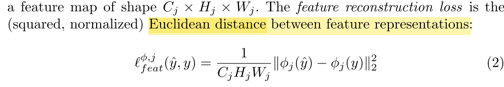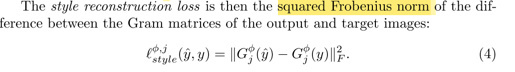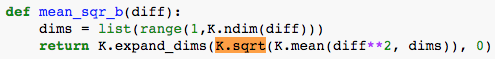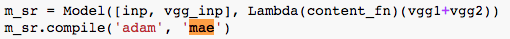# Lesson 10 Discussion

Right - keras will handle that averaging when it does the mse or mae calculation.

1 Like

Thanks - how many did you get doing it that way? It’s much better than the manual approach I used!

0 Likes

(kelvin) #23

I get the same # of nouns but more synset matches.

0 Likes

(Thundering Typhoons) #24

I won’t be able to train Devise on the entire imagenet because of hardware limitation. Can Jeremy (or somebody else) share the pretrained models? It seems pretty useful for a large number of applications.

1 Like

Pretrained model weights
(alenavkruchkova) #25

I wonder if anyone has experience deploying models? there is a thread Deploying models in production but I have not yet neeb able to get my questions answered

0 Likes

We do plan to release this, and other pre-trained models - might not be for a while though since I need to look at what needs to be included, and how to distribute.

3 Likes

Pretrained model weights
(Gidi Shperber) #27

Hi, I have a few questions re the DCGAN:

• what is the meaning of mode=2 in BatchNormalization? i didn’t find it on keras docs…
• what is the meaning of upsampling?
• the results doesnt look so good, while in the papers they look better… why is that so? will more training help?
• It was said in the lecture that the difference between the standard GAN an the WGAN is small - only changing the loss function. can I do this change in the keras-DCGAN notebook and convert it to WGAN? i’ve tried to write a custom loss function like so to start with,

def custom_loss(y_true, y_pred):
y_pred = K.clip(y_pred, epsilon, 1.0-epsilon)
out = -(y_true * K.log(y_pred) + (1.0 - y_true) * K.log(1.0 - y_pred))
return K.mean(out, axis=-1)

and use it with

but it didn’t seem to work…

0 Likes

(Constantin) #28

Is there something like model.summary() in pyTorch? I couldn’t find it on the documentation. I tried model.type, which gives you something like (see below). But I am missing the info on the shape of each output, like (None, 28, 28, 512) or similar. Any ideas?

``````  (main): Sequential (
(initial-100.512.convt): ConvTranspose2d(100, 512, kernel_size=(4, 4), stride=(1, 1), bias=False)
(initial-512.batchnorm): BatchNorm2d(512, eps=1e-05, momentum=0.1, affine=True)
(initial-512.relu): ReLU (inplace)
(pyramid-512.256.convt): ConvTranspose2d(512, 256, kernel_size=(4, 4), stride=(2, 2), padding=(1, 1), bias=False)
(pyramid-256.batchnorm): BatchNorm2d(256, eps=1e-05, momentum=0.1, affine=True)
(pyramid-256.relu): ReLU (inplace)
(pyramid-256.128.convt): ConvTranspose2d(256, 128, kernel_size=(4, 4), stride=(2, 2), padding=(1, 1), bias=False)
(pyramid-128.batchnorm): BatchNorm2d(128, eps=1e-05, momentum=0.1, affine=True)
(pyramid-128.relu): ReLU (inplace)
(pyramid-128.64.convt): ConvTranspose2d(128, 64, kernel_size=(4, 4), stride=(2, 2), padding=(1, 1), bias=False)
(pyramid-64.batchnorm): BatchNorm2d(64, eps=1e-05, momentum=0.1, affine=True)
(pyramid-64.relu): ReLU (inplace)
(extra-0-64.64.convt): ConvTranspose2d(64, 64, kernel_size=(3, 3), stride=(1, 1), padding=(1, 1), bias=False)
(extra-0-64.batchnorm): BatchNorm2d(64, eps=1e-05, momentum=0.1, affine=True)
(extra-0-64.relu): ReLU (inplace)
(final.64-3.convt): ConvTranspose2d(64, 3, kernel_size=(4, 4), stride=(2, 2), padding=(1, 1), bias=False)
(final.3.tanh): Tanh ()
)
)>
``````
0 Likes

(David Gutman) #29

They’ve removed `mode` from the BatchNormalization layer in Keras.

1 Like

(Constantin) #30

I found if you go

``````for p in model.parameters(): print(p.data);
``````

you get a printout of the parameters (all weights, X_train and y_train) which live in the GPU. Pretty cool, pyTorch.
Still, not quite what I wanted.

1 Like

(Brendan Fortuner) #31

Is anyone seeing 4-6x speedups after installing pillow-simd? I’m not and wondering why.

I get similar runtimes for pillow and pillow-simd–about 35 microseconds for the following code:

``````import PIL
print (PIL.PILLOW_VERSION) #4.0.0.post0 (simd) or 4.0.0 (pillow)
img = Image.open(fnames)
%timeit img.resize((224,224))
``````

Here’s how I installed pillow-simd:

``````pip uninstall pillow
CC="cc -mavx2" pip install -U --force-reinstall pillow-simd
``````

Any thoughts?

0 Likes

(Kent) #32

One question for the neural-sr notebook. In the below code, I think the intention is to use preproc Lambda function as the input into VGG16, so that input can be preprocessed prior to VGG layers.

``````vgg_inp=Input(shp)
vgg= VGG16(include_top=False, input_tensor=Lambda(preproc)(vgg_inp))
``````

However, in keras.applicaitons.vgg16.VGG16 source code (around line 125), I found the below lines:

``````if input_tensor is not None:
inputs = get_source_inputs(input_tensor)
``````

What get_source_inputs function does is to trace back from the input tensor back to the original input, which is vgg_inp in this case. As a result, the VGG model created is actually using vgg_inp rather than using the preproc input.

I am wondering whether I understood this correctly here?

I also generated a visualized model, and from the graph, I don’t see the preproc input either.

You can find the full visualized model here: https://drive.google.com/file/d/0BzfDHLmb4-cfZG1sSFRZYmdqTDQ/view?usp=sharing . The preview is a bit messy but you should be able to read. Or, you can download the file and open it with a browser for a nicer view. The above-mentioned layers are near the end (i.e. inputs to model_2, here no preproc is shown).

2 Likes

(Constantin) #33

I seem to be missing something down the line of WGAN loss functions: The two main changes to convert a DCGAN into WGAN are

1. Change loss function to mse

2. Clip weight parameters in netD to the interval [-0.01, 0.01]

What I don’t understand is at which point you change the loss function from crossentropy to mse?
Is it for the generator G or the discriminator D or the whole model M? Or combinations thereof? My understanding of pytorch so far didn’t allow me to read it from the source code of WGAN.ipynb / dcgan.py.

0 Likes

(Kent) #34

One more question regarding notebook neural-sr:

In the paper, it mentions that the loss function is “Euclidean distance between feature representations”, or “squared Frobenius norm”. Basically that means the loss function is the squared differences (rather than square root)Is there a reason why in our notebook we are using square root of the mean square differences:Since later we are using ‘mae’ rather than ‘mse’ as the optimizer, it means that we never used the “square” version of the calculation as proposed in the paper.I am confused here when to use the square version, and when to use the square root version? Obviously, the square version of the loss function produces a much larger loss (in the magnitude of tens of millions).

1 Like

(Constantin) #35

I can’t quite get one difference in the training procedure of DCGAN vs. WGAN:
In DCGAN you train

``````gl.append(m.train_on_batch(noise(bs), np.zeros([bs])))
``````

with m being `Model([generator, discriminator])`
So, you train the generator through the whole model.

In WGAN you train first the discriminator for a couple of epochs and then you train the generator directly

``````            make_trainable(netD, False)
errG = step_D(netG(create_noise(bs)), one)
optimizerG.step()
gen_iterations += 1
``````

What does this difference come from?

1 Like

They’re the same - in the keras dcgan the discriminator is first set to non-trainable.

0 Likes

No reason - although I doubt it makes any significant difference. Feel free to try removing it and let us know if you find any impact!0 Likes

Well that’s intriguing! Do you want to see if you can come up with an approach that works correctly, and see what (if any) impact it has?

0 Likes

(Kent) #39

I actually tried, and the square version didn’t train. The square root version does. I am wondering why1 Like